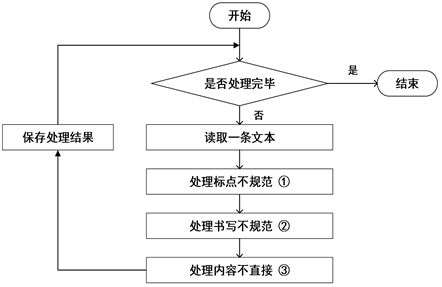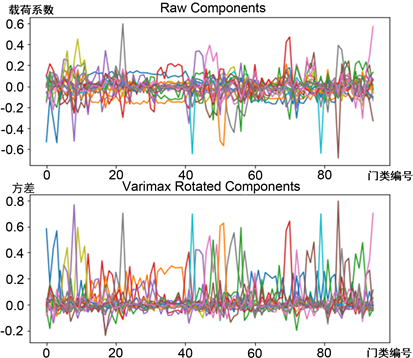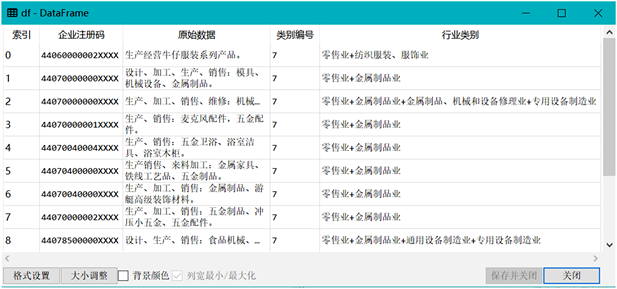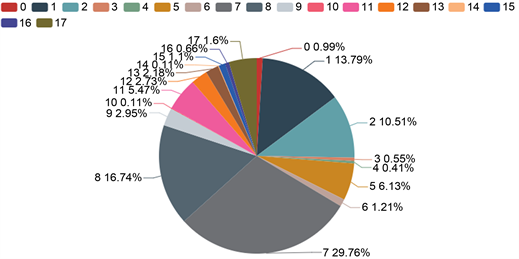﻿ 基于因子分析和K-means聚类算法的行业聚类研究

基于因子分析和K-means聚类算法的行业聚类研究Research on Industry Clustering Based on Factor Analysis and K-Means Clustering Algorithm

Abstract: The business scope of the enterprise in the industrial and commercial registration information records the main production and operation activities of the enterprise, which is an important standard to reflect the industry category of the enterprise. Industry clustering is not only convenient for the state to manage enterprises, but also conducive to the positioning of enterprises and the development of economy in line with the national trend. In this paper, based on factor analysis and K-means clustering algorithm, and taking the national economic industry classification as the standard text, this paper conducts industry cluster analysis on enterprise business field samples. Firstly, the optimal number of K-means clustering is obtained by factor analysis algorithm, and then the business scope of enterprises is clustered by K-means algorithm, and the industry category of each enterprise is obtained. Finally, the clustering results are evaluated by artificial evaluation and Davies Bouldin index (DBI) to prove the effectiveness of the method.

1. 引言

2. 相关工作

2.1. 国民经济行业分类

《国民经济行业分类》是指由国家统一制定的，按照生产的同一性，对于一个国家的国民经济的所有生产活动进行生产性质归属性分组而形成的规范标准 。具有国家强制推行的标准化特征 。具体内容如表1所示。

《国民经济行业分类》规定了全社会经济活动的分类与代码，能够满足国家在统计、计划、税收、工商等宏观管理中对经济活动的分类，也可用于信息处理和信息交换。Table 1. Classification and code table of national economic sectors

2.2. 待聚类文本预处理

2.2.1. 文本来源及特点Table 2. Business scope of some enterprises

1) 内容不多：每条信息代表一个企业的经营范围，字数大概在几个字至几百字间。

2) 标点不规范：主要体现为中英文状态下标点符号的不准确使用。

3) 书写不规范：主要体现为“规则组合(如：加工、销售：机制沙)”和“非规则组合(如：城市建设管理咨询、代理；城市环境卫生维护、道路清扫)”。

4) 内容不直接：主要体现为“凭有效的《食品生产许可证》经营”等信息。

2.2.2. 文本异常值处理Figure 1. Pretreatment process

2.3. 中文分词的“归一化”处理

2.4. 建立聚类数据集

3. 因子分析抽取特征

$\begin{array}{l}{X}_{1}={a}_{11}{F}_{1}+{a}_{12}{F}_{2}+\cdots +{a}_{1n}{F}_{n}+{e}_{1}\\ {X}_{2}={a}_{21}{F}_{1}+{a}_{22}{F}_{2}+\cdots +{a}_{2n}{F}_{n}+{e}_{2}\\ \text{\hspace{0.17em}}\text{\hspace{0.17em}}\text{\hspace{0.17em}}\text{\hspace{0.17em}}\text{\hspace{0.17em}}\text{\hspace{0.17em}}\text{\hspace{0.17em}}\text{\hspace{0.17em}}\text{\hspace{0.17em}}\text{\hspace{0.17em}}\text{\hspace{0.17em}}\text{\hspace{0.17em}}\text{\hspace{0.17em}}\text{\hspace{0.17em}}\text{\hspace{0.17em}}\text{\hspace{0.17em}}\text{\hspace{0.17em}}\text{\hspace{0.17em}}\text{\hspace{0.17em}}\text{\hspace{0.17em}}\text{\hspace{0.17em}}⋮\\ {X}_{m}={a}_{m1}{F}_{1}+{a}_{m2}{F}_{2}+\cdots +{a}_{mn}{F}_{n}+{e}_{m}\end{array}$ (1)

$a=\left({a}_{11},{a}_{12},\cdots ,{a}_{1n},\cdots ,{a}_{m1},{a}_{m2},\cdots ,{a}_{mn}\right)$ 代表参数，即变量之间的相关系数，值越大，相关性越大；

$F=\left({F}_{1},{F}_{2},{F}_{3},\cdots ,{F}_{n}\right)$ 代表公共(共性)因子，简称因子；

$e=\left({e}_{1},{e}_{2},{e}_{3},\cdots ,{e}_{m}\right)$ 代表特殊因子，是不可直接观测的数据，在分析中一般省略 。

3.1. 主成分法提取因子

$X=\left(\begin{array}{cccc}{x}_{11}& {x}_{12}& \cdots & {x}_{1n}\\ {x}_{21}& {x}_{22}& \cdots & {x}_{2n}\\ ⋮& ⋮& \ddots & ⋮\\ {x}_{m1}& {x}_{m2}& \cdots & {x}_{mn}\end{array}\right)$ (2)

${x}_{ij}^{*}=\frac{{x}_{ij}-{\stackrel{¯}{x}}_{j}}{\sqrt{\mathrm{var}\left({x}_{j}\right)}}\text{\hspace{0.17em}}\text{\hspace{0.17em}}\left(i=1,2,3,\cdots ,m;j=1,2,\cdots ,n\right)$ (3)

${\stackrel{¯}{x}}_{j}=\frac{1}{m}\underset{i=1}{\overset{m}{\sum }}{x}_{ij}\text{\hspace{0.17em}}\text{\hspace{0.17em}}\left(i=1,2,3,\cdots ,m;j=1,2,\cdots ,n\right)$ (4)

$\mathrm{var}\left({x}_{j}\right)=\frac{1}{m-1}\underset{i=1}{\overset{m}{\sum }}{\left({x}_{ij}-{\stackrel{¯}{x}}_{j}\right)}^{2}\text{\hspace{0.17em}}\text{\hspace{0.17em}}\left(j=1,2,3,\cdots ,n\right)$ (5)

$A=\left(\begin{array}{cccc}{a}_{11}& {a}_{12}& \cdots & {a}_{1n}\\ {a}_{21}& {a}_{22}& \cdots & {a}_{2n}\\ ⋮& ⋮& \ddots & ⋮\\ {a}_{n1}& {a}_{n2}& \cdots & {a}_{nn}\end{array}\right)$ (6)

${a}_{ij}=\frac{1}{m-1}\underset{l=1}{\overset{m}{\sum }}{x}_{li}{x}_{lj}\text{\hspace{0.17em}}\text{\hspace{0.17em}}\left(i,j=1,2,3,\cdots ,n\right)$ (7)

$C=\frac{{g}_{i}}{\underset{i=1}{\overset{n}{\sum }}{g}_{i}}$ (8)

Table 5. Variance, proportion and cumulative rate of eigenvalues of correlation coefficient (P = 18)

3.2. 因子旋转

${g}_{j}^{2}=\underset{i=1}{\overset{m}{\sum }}{a}_{ij}^{2}$ (9)Figure 2. Factor analysis results

① Raw Components (主成分分析图)：该图峰值围绕0.0的中轴线上下浮动，每个峰值表示每个主成分在每个变量上的权重值大小，但峰值比较不够明显。

② Varimax Rotated Components (因子旋转图)：该图峰值集中围绕在0.0中轴线以上，且每个峰值大的越大，小的越小，可较清楚地看出峰值间的差别。

4. 聚类实现过程与结果

K-means聚类算法是一个迭代的过程 ，其具体步骤如下：

1) 在样本中选取K个点作为初始质心，即每个K代表一个聚类中心；

2) 对每个样本点，本文通过欧式距离计算方式按照距离最近的原则将每个数据点划分到离它最近的聚类中心K所对应的类别中 ；

3) 经过步骤2)后，形成了K个集合，即K个类别，然后重新计算每个类别的质心，更新聚类中心的位置；

4) 在3)中，如果新质心和旧质心间的距离小于某一阈值，则判断达到预期效果，算法终止，否则迭代2)~3)步骤 。Figure 3. Data category display

5. 评论与分析Figure 4. Cluster analysis results

$DBI=\frac{1}{k}\underset{i=1}{\overset{k}{\sum }}\underset{i\ne j}{\mathrm{max}}\left(\frac{avg\left({S}_{i}\right)+avg\left({S}_{j}\right)}{dist\left({\omega }_{i},{\omega }_{j}\right)}\right)$ (10)Table 6. Artificial evaluation by factor analysis

6. 结束语

 陈正伟. 国民经济行业分类及应用[Z]. 重庆: 重庆工商大学, 2014.

 吴娇. 四川省各市州经济综合发展水平比较研究——基于因子分析和K-means聚类分析[J]. 知行铜仁, 2019(3): 35-39.

 彭凯, 秦永彬, 许道云. 应用因子分析和K-MEANS聚类的客户分群建模[J]. 计算机科学, 2011, 38(5): 154-158, 198.

 黎明, 熊伟. 基于因子分析与聚类分析的化妆品上市公司绩效评价[J]. 财会通讯, 2020(14): 96-99.

 任恒妮. 大数据K-means聚类算法的研究与应用[J]. 信息技术, 2019, 43(11): 20-23.

 王春枝. 因子分析中公因子提取方法的比较与选择[J]. 内蒙古财经学院学报(综合版), 2014, 12(1): 90-94.

 Martinez-Martin, P., Rojo-Abuín, J.M., Weintraub, D., Chaudhuri, K.R., Rodriguez-Blázquez, C., Rizos, A. and Schrag, A. (2020) Factor Analysis and Clustering of the Movement Disorder Society-Non-Motor Rating Scale. Movement Disorders, 35, No. 6.
https://doi.org/10.1002/mds.28002

 韩雪, 张业, 朱聪慧. 企业经营范围文本自动分类方法探究[J]. 标准科学, 2012(1): 93-96.

 Martinez-Martin, P., Rojo-Abuín, J.M., Weintraub, D., Chaudhuri, K.R., Rodriguez-Blázquez, C., Rizos, A. and Schrag, A. (2020) Factor Analysis and Clustering of the Movement Disorder Society-Non-Motor Rating Scale. Movement Disorders, 35, 969-975.

 Subramaniyam, B.A., Muliyala, K.P., Suchandra, H.H. and Reddi, V.S.K. (2020) Diagnosing Catatonia and Its Dimen-sions: Cluster Analysis and Factor Solution Using the Bush Francis Catatonia Rating Scale (BFCRS). Asian Journal of Psychiatry, 52, 102002.
https://doi.org/10.1016/j.ajp.2020.102002

 Wen, F., Du, H., Ding, L., Hu, J., Huang, Z., Huang, H., et al. (2020) Clinical Efficacy and Safety of Drug Interventions for Primary and Secondary Prevention of Osteoporotic Fractures in Postmenopausal Women: Network Meta-Analysis Followed by Factor and Cluster Analysis. PLoS ONE, 15, e0234123.
https://doi.org/10.1371/journal.pone.0234123

 秦志勇. 安徽省医疗卫生机构服务水平综合评价——基于因子分析和聚类分析方法[J]. 合肥学院学报(综合版), 2020, 37(2): 63-68.

 Zhang, Q.H. (2019) Customers Segmentation Based on Factor Analysis and Cluster. E-Commerce Letters, 8, 53-62.

 Wang, W. (2017) Stock Evaluation Based on Factor Analysis and Cluster-ing. Chongqing Technology and Business University. In: Proceedings of 2017 2nd International Seminar on Education Innovation and Economic Management (SEIEM 2017), Atlantis Press, 473-476.
https://doi.org/10.2991/seiem-17.2018.118

 金涛, 戴玉刚. 浅析文本聚类有效性评价的方法[J]. 中文信息, 2018(5): 3.

 黄越辉, 曲凯, 李驰, 司刚全. 基于K-means MCMC算法的中长期风电时间序列建模方法研究[J]. 电网技术, 2019, 43(7): 2469-2476.

Top